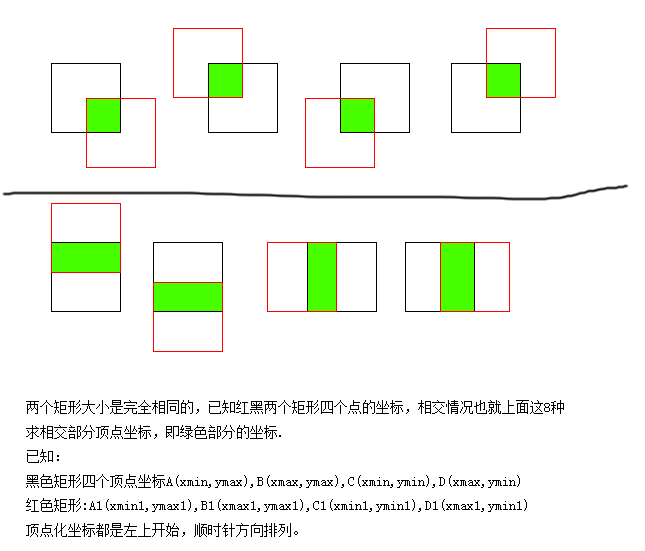# 两个矩形相交的算法求助...全文
439 2 打赏 收藏 举报

2 条回复

• 打赏
• 举报

• 打赏
• 举报

C_xmin = Math.max(A_xmin,B_xmin) C_xmax = Math.min(A_xmax,B_xmax) C_ymin = Math.max(A_ymin,B_ymin) C_ymax = Math.min(A_ymax,B_ymax)判断两个矩形相交以及求出相交的区域判断矩形相交以及求出相交的区域